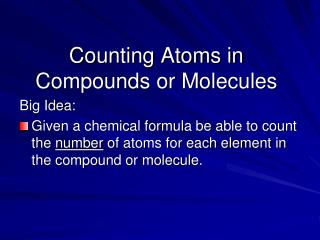DownloadDownload PresentationBig Idea:

# Big Idea:

Télécharger la présentation## Big Idea:

- - - - - - - - - - - - - - - - - - - - - - - - - - - E N D - - - - - - - - - - - - - - - - - - - - - - - - - - -
##### Presentation Transcript

1. Counting Atoms in Compounds or Molecules Big Idea: • Given a chemical formula be able to count the number of atoms for each element in the compound or molecule.

2. Parts of a Chemical Formula Element Symbol 2 H2O 4 Pb(OH)4 Subscript Coefficient

3. 3 Parts of a Chemical Formula 1. Chemical symbols - Written in the order they appear on the periodic table, from left to right. 2. The subscript - Gives the number of atoms - Must be written after the symbol, half size 3. The coefficient - Gives the number of compounds or molecules • Must be written regular size before the formula

4. Counting Atoms • Rules for counting atoms in a molecule or compound. • A subscript shows how many atoms of that element are bonded in the molecule. • If there is no subscript then one atom of the element is present. Group all like atoms!! • If the subscript is outside the brackets, all the atoms inside the brackets are multiplied by the subscript outside the brackets. • Coefficients are multiplied last. The total number of atoms in the formula is multiplied by the coefficient.

5. Count the Atoms • Oxygen – 1 atom • Hydrogen – 2 atoms • Formula - H2O

6. Count the Atoms • Calcium – 1 atom • Oxygen – 2 atoms • Hydrogen – 2 atoms Ca(OH)2

7. Coefficients The number of water molecules is shown with a coefficient. Coefficients are written in front of the chemical formula. 3 H2O

8. Count all the atoms 3 H2O The coefficient multiplies all the atoms after it. Hydrogen 3 x 2 = 6 Oxygen 3 x 1 = 3

9. Test Yourself lead 1 Oxygen 4 Hydrogen 4

10. Counting atoms lead 1 Oxygen 4 Hydrogen 4 magnesium 3 phosphorus 2 oxygen 6 arsenic 1 iodine oxygen 20 5 copper 2 8 4 oxygen chlorine

11. Try Grouping Like Atoms • Given the formula CH3O(CH2)2OH • How many atoms of carbon in the formula? • 3 • How many atoms of oxygen in the formula? • 2 • How many atoms of hydrogen in the formula? • 8